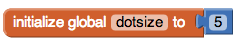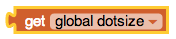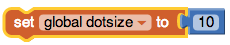# 3.2. Paint Pot Tutorial¶

## 3.2.1. Introduction and Goals¶

 Paint Pot is a “finger painting” app. It simulates dipping your finger in a pot of a paint then drawing with it on a canvas. The app uses buttons to change the paint colors and MIT App Inventor’s touch and drag event handlers to draw circles and lines on the canvas. (TeacherTube Version) Learning Objectives: I will learn to follow a tutorial to create the PaintPot app deepen my understanding of event-driven programming learn how to use a variable to make a program more general Language Objectives: I will be able to explain the result of programming statements that include variables and assignments describe how a global variable is an abstraction use target vocabulary, such as variable, assignment, expression, operator, and pseudocode while describing app features and User Interface with the support of concept definitions and vocabulary notes from this lesson

## 3.2.2. Learning Activities¶

### Tutorial Part 1: Painting and Drawing on a Canvas

To get started, open MIT App Inventor with the Paint Pot Template in a separate tab and follow along with the following video or your teacher.

### Tutorial Part 2: Varying the Size of the Dots

Watch the video tutorial below or click here for the text version of the tutorial.
(TeacherTube version)

### AP CSP Pseudocode: Variables and Assignment Statements

A variable provides a way to name a memory location in your program to hold different values. It is a data abstraction that exists in all programming languages. In MIT App Inventor, we set up a variable using the initialize global variable block. The get block is used to get the variable's current value whenever needed in the program. The set block is used to assign or change the value of the variable.The AP CS Principles Exam does not have a designated programming language. It uses pseudocode which is a cross between computer code and everyday English. Pseudocode is less precise than actual computer code, such as Java or Python or MIT App Inventor, but more precise and less wordy than everyday English. The AP CSP Reference Sheet is provided during the exam to help you understand the AP pseudocode format used in the exam. It includes two pseudocode styles: text-based and block-based.

In the College Board AP CSP exam, MIT App Inventor set blocks are called assignment statements and are represented as the left-pointing arrow (←). The following text and block style pseudocode is used to assign values to variables:

FunctionAP Text AP Block MIT App Inventor
Assignment:a ← expression
a ← expressionThe DISPLAY statement is used to display variable values or the value resulting from mathematical expressions like (a+b)/2. Expressions are made up of values, variables, math operators, and sometimes mathematical procedures like getting a random number or squaring a number. In mathematical expressions, the operators * for multiplication, / for division, and the mod operator (remainder after division) are done before + and - like in math, unless there are parentheses that for example tell the computer to do (a+b) first before dividing by 2. Notice that * is used for multiplication instead of x, because x would get confused with a variable name. Expressions are evaluated to produce a single value.

FunctionAP Text AP Block
Display:DISPLAY(expression)
DISPLAY expression
Expressions:a + b, a - b, a * b, a/b
a + b

For example, here is AP style pseudocode to set the dotsize variable to 5 and then increment it (add one to it). The variable dotsize will have the value 6 after these two lines of code are executed. The value stored in a variable will be the most recent value assigned.

FunctionAP Text AP Block MIT App Inventor
Set dotsize to 5dotsize ← 5
dotsize ← 5Increment dotsize dotsize ← dotsize + 1
dotsize ← dotsize + 1Some exercises involving AP pseudocode for assignment are below. More complex AP pseudocode will be shown in Unit 4.

## 3.2.3. Summary¶

In this lesson, you learned how to:

## 3.2.4. Self-Check¶

### Vocabulary

Here is a table of some of the technical terms we've introduced in this lesson. Hover over the terms to review the definitions.

 variable assignment expression operator pseudocode